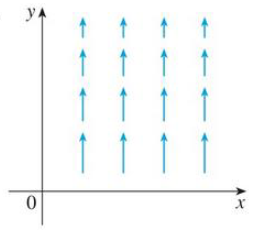Chapter 16.5, Problem 9E

Chapter
Section
Textbook Problem

The vector field F is shown in the xy-plane and looks the same in all other horizontal planes. (In other words, F is independent of z and its z-component is 0.)(a) Is div F positive, negative, or zero? Explain.(b) Determine whether curl F = 0. If not, in which direction does curl F point?9.(a)

To determine

To find and explain: The div F.

Explanation

Formula used:

Consider the standard equation of a divergence of vector field for F=Pi+Qj+Rk

divF=Px+Qy+Rz (1)

As F is independent of z-component (R=0) is Rx=Ry=Rz=0 .

From Figure, the x-component of each vector is 0 (P=0) , hence Px=Py=Pz=0

and Q dec

(b)

To determine

Whether curl F=0 or not.

Still sussing out bartleby?

Check out a sample textbook solution.

See a sample solution

The Solution to Your Study Problems

Bartleby provides explanations to thousands of textbook problems written by our experts, many with advanced degrees!

Get Started

Convert the expressions in Exercises 6584 to power form. 35x5x8+72x3

Finite Mathematics and Applied Calculus (MindTap Course List)

Find the derivative of the function. r(t)=10t2

Single Variable Calculus: Early Transcendentals, Volume I

If f(x)=lnx+tan1x, find (f1)(/4).

Calculus (MindTap Course List)

Evaluate.

Mathematical Applications for the Management, Life, and Social Sciences

Show that ddx arctan(tanh x) = sech 2x.

Single Variable Calculus: Early Transcendentals

The slope of the tangent line to r = cos θ at is:

Study Guide for Stewart's Multivariable Calculus, 8th

An estimate of 13x4 using Simpsons Rule with n = 4 gives: a) 2425 b) 1483 c) 1523 d) 2445

Study Guide for Stewart's Single Variable Calculus: Early Transcendentals, 8th